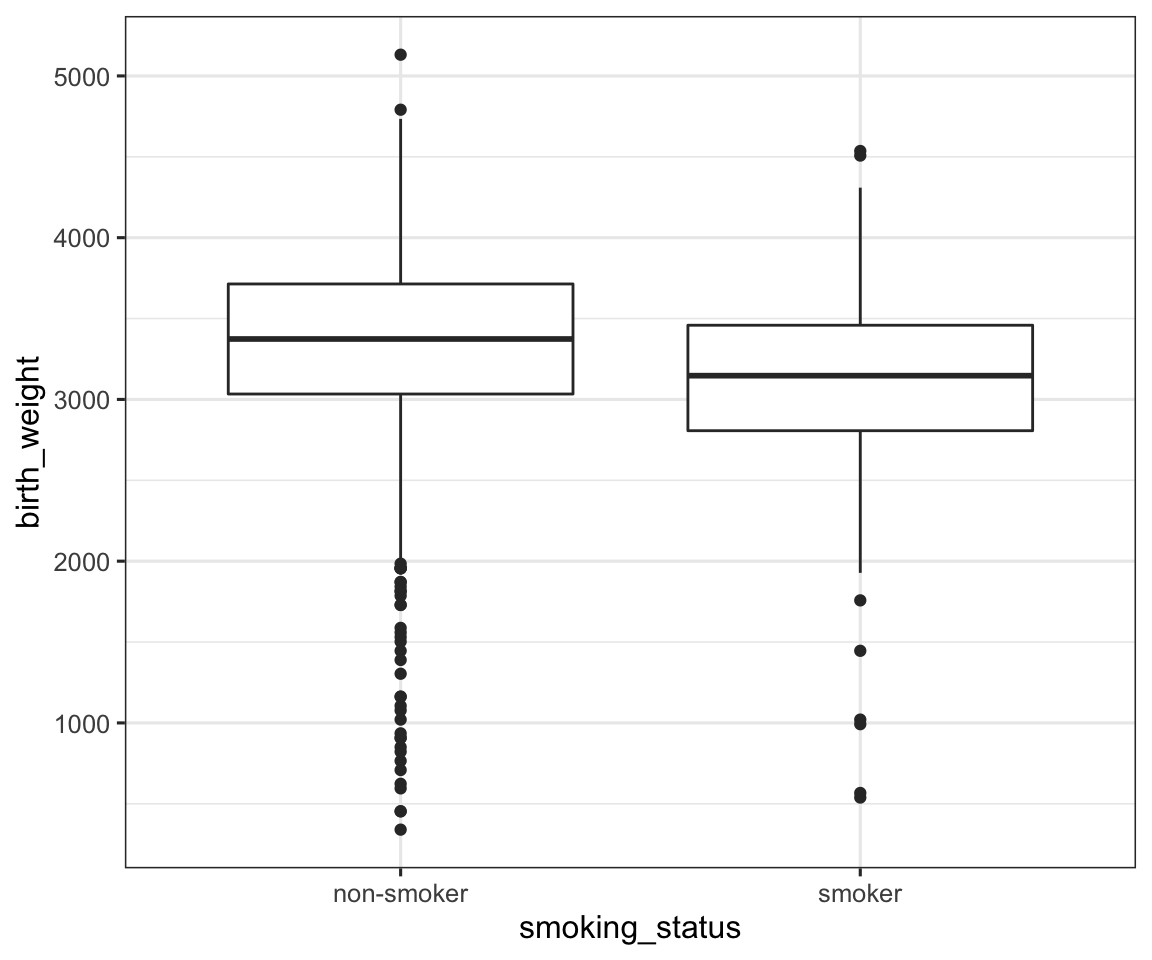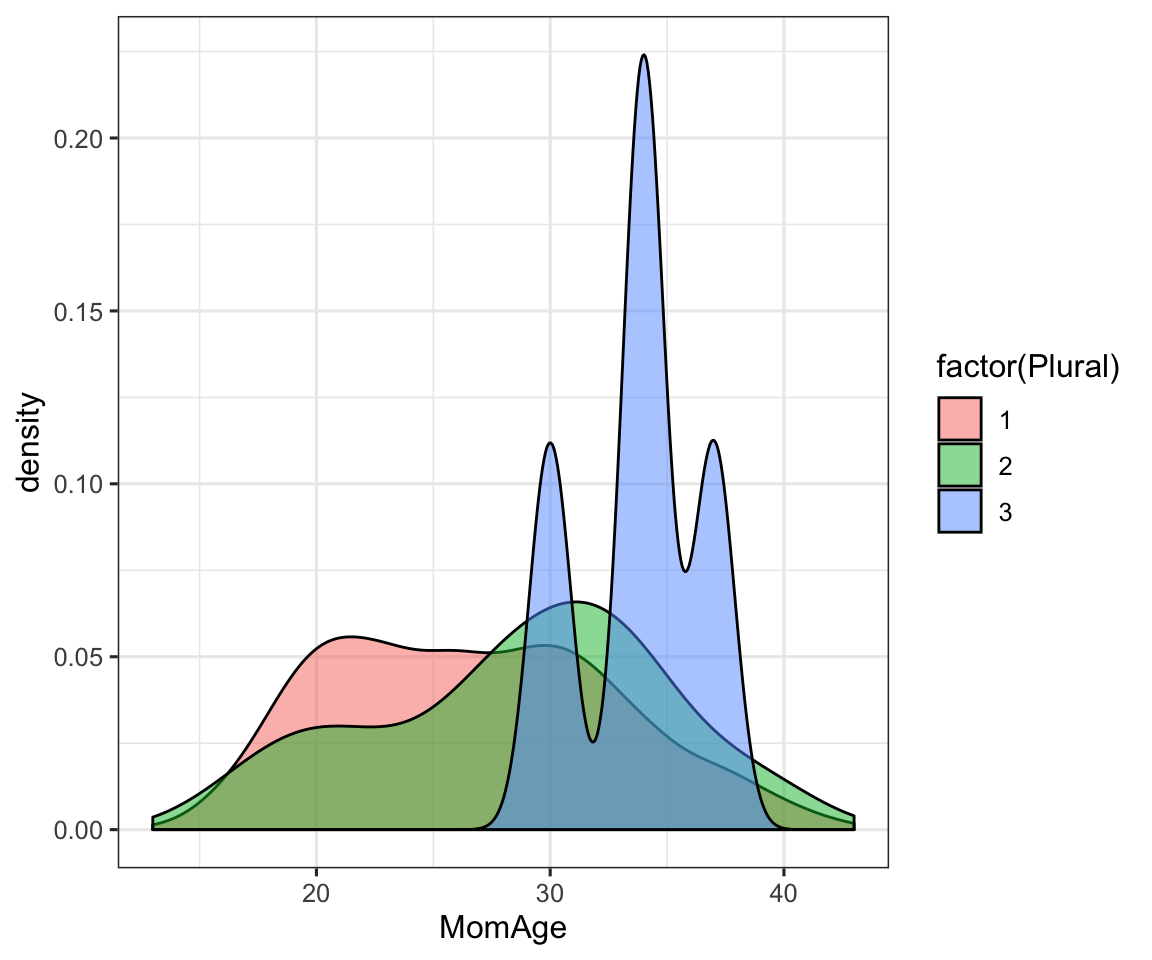## Project 1 Example solution

Claus O. Wilke, EID

This is the dataset you will be working with:

NCbirths <- read_csv("https://wilkelab.org/classes/SDS348/data_sets/NCbirths.csv")
## Parsed with column specification:
## cols(
##   Plural = col_double(),
##   Sex = col_double(),
##   MomAge = col_double(),
##   Weeks = col_double(),
##   Gained = col_double(),
##   Smoke = col_double(),
##   BirthWeightGm = col_double(),
##   Low = col_double(),
##   Premie = col_double(),
##   Marital = col_double()
## )

### Part 1

Question: Is there a relationship between whether a mother smokes or not and her baby’s weight at birth?

To answer this question, first calculate the mean birthweight of babies to mothers who smoke and don’t smoke. Then run a t-test to test for significant differences. Finally, using ggplot, make a graph that shows birth weight vs. smoking status, plotted as a boxplot.

Introduction: We are working with the NCbirths dataset, which contains 1409 birth records from North Carolina in 2001. In this dataset, each row corresponds to one birth, and there are ten columns providing information about the birth, the mother, and the baby. Information about the birth includes whether it is a single, twin, or triplet birth, the number of completed weeks of gestation, and whether the birth is premature. Information about the baby includes the sex, the weight at birth, and whether the birth weight should be considered low. Information about the mother includes her age, the weight gained during pregnancy, whether she is a smoker, and whether she is married.

To answer the question of Part 1, we will need to work with two variables, the baby’s birthweight (column BirthWeightGm) and the mother’s smoking status (column Smoke). The birthweight is provided as a numeric value, in grams. The smoking status is encoded as 0/1, where 0 means the mother is not a smoker and 1 means she is a smoker.

Approach: Our approach will be to first subdivide the dataset into two subsets, smoker and non-smoker moms, using the function filter(). We then use mutate() to add a new variable smoking_status with values "smoker" and "non-smoker", because it’s always better to encode categories in words than as 0/1. Finally, we bind the two datasets together using rbind(). Then we can use group_by() and summarize() to calculate the mean birthweight for each group.

To perform the t-test, we use the function t.test(), and take advantage of the fact that we have previously subdivided the data into two subsets.

To plot, we use geom_boxplot(), mapping smoking status onto the x axis and birthweight onto the y axis. Boxplots are a good choice to visualize how numerical values (here, birthweight) are distributed across differen categories (here, smoking status) (https://serialmentor.com/dataviz/boxplots-violins.html).

Analysis: First we subdivide the data into two groups, combine back together, and calculate summary statistics.

# first the data for ownly the smokers
smokers <-
NCbirths %>%
filter(Smoke == 1) %>%
select(birth_weight = BirthWeightGm) %>% # discard irrelevant columns to keep dataset clean
mutate(smoking_status = "smoker") # add new column that spells out "smoker" instead of numerical 1

# now the same for non-smokers
non_smokers <-
NCbirths %>%
filter(Smoke == 0) %>%
select(birth_weight = BirthWeightGm) %>%
mutate(smoking_status = "non-smoker")

# combine the two data frames together into one
smoking_bw <- rbind(smokers, non_smokers)

# now we can group and summarize
smoking_bw %>%
group_by(smoking_status) %>%
summarize(mean_birth_weight = mean(birth_weight))
## # A tibble: 2 x 2
##   smoking_status mean_birth_weight
##   <chr>                      <dbl>
## 1 non-smoker                 3336.
## 2 smoker                     3098.

The mean birth weight of babies from mothers who don’t smoke is 3336 grams, and the mean birth weight of babies from mothers who do smoke is 3098 grams. Next we do a t test to test whether this difference is significant.

t.test(smokers$birth_weight, non_smokers$birth_weight)
##
##  Welch Two Sample t-test
##
## data:  smokers$birth_weight and non_smokers$birth_weight
## t = -5.1072, df = 281.63, p-value = 6.035e-07
## alternative hypothesis: true difference in means is not equal to 0
## 95 percent confidence interval:
##  -329.1170 -145.9965
## sample estimates:
## mean of x mean of y
##  3098.270  3335.826

There is a significant difference ($$P<10^{-6}$$). Mothers who smoke have babies that weight less than do mothers who don’t smoke.

Finally, we make our plot.

ggplot(smoking_bw, aes(smoking_status, birth_weight)) +
geom_boxplot()Discussion: The babies of mothers who smoke weigh significantly less than the babies of mothers who don’t smoke. Thus, smoking has a negative effect on the baby’s growth during gestation. However, this effect is relatively modest (the difference in means is a little over 200 grams) compared to the wide range of birth weights observed in this data set (from less than 500 grams to over 5000 grams), as shown by the boxplot. The boxplot further shows that the variation in birth weight is not or only minimally affected by smoking status. The heights of the two boxes look nearly identical, and both the largest and smallest birthweight in either group is close to the respective extreme value in the other group.

Since the range of observed birth weights is so large, and not seemingly affected by smoking status, it might be interesting to do further analysis on the causes. For example, we would expect premature births to be particularly light, and also babies that are part of twin or triplet births.

### Part 2

Question: How are the ages of mothers in this dataset distributed, and do they differ among mothers with single, twin, or triplet births?

Introduction: We continue to work with the same dataset. For this analysis, we will work with the data columns Plural and MomAge. Plural indicates whether a birth was single (1), twin (2), or triplet (3). MomAge provides the age of the mother, in years.

Approach: To obtain a rough outline of how the mothers’ ages are distributed, we first calculate the minimum, medium, and maximum age, as well as the interquartile range (IQR), separately for mothers with single, twin, and triplet births. We do this by first grouping by the Plural column and then using summarize() with the functions min(), median(), max(), and IQR() to obtain the desired summary statistics. We also count how many mothers are present in each category, since likely there are many more mothers with single births than with triplet births. Unlike in Part 1, we leave the Plural column as is and don’t convert it into words (“single”, “twin”, “tripple”), because the numbers make sense as count values (1, 2, 3 children born at once).

For visualization, we will make a density plot, using geom_density(). Density plots are a good choice to visualize how quantities are distributed (https://serialmentor.com/dataviz/histograms-density-plots.html). We will fill the densities according to the variable Plural, so that we obtain three separate age distributions, one for single births, one for twin births, and one for triplet births.

Analysis: We begin with the summary statistics.

NCbirths %>%
group_by(Plural) %>%
summarize(
count = n(),
min = min(MomAge),
median = median(MomAge),
max = max(MomAge),
iqr = IQR(MomAge)
)
## # A tibble: 3 x 6
##   Plural count   min median   max   iqr
##    <dbl> <int> <dbl>  <dbl> <dbl> <dbl>
## 1      1  1362    13     26    43  9
## 2      2    43    16     30    40  8
## 3      3     4    30     34    37  1.75

The mothers’ ages in this dataset range from 13 to 43. The widest age range is observed for mothers with single births. Mothers of triplets span a much narrower age range from 30 to 37. Notably, there are only 4 mothers of triplets but 1362 mothers of single babies.

# we need to fill by factor(Plural) instead of Plural to make sure
# ggplot treats this variable as categorical, not numerical.
ggplot(NCbirths, aes(MomAge, fill = factor(Plural))) +
geom_density(alpha = 0.5)Discussion: The most notable feature of this dataset is difference in the number of mothers with singlet, twin, and triplet births. There are 1362 of the first, but only 43 of the second and only 4 of the third type. Consequently, we expect the age range to be the broadest for singlet births, simply we have so many more observations, and indeed the minimum and maximum ages are the most extreme for mothers with single births. The IQR is also the largest in this case. Next, we see that the median age seems to increase from singlet to twin to triplet (26, 30, and 34 years, respectively), but we did not perform any statistical test to see whether this pattern is significant. Notably, the four mothers with triplets are all comparatively old, between 30 and 37 years, with an IQR of 1.75 years. We can speculate about the origin of this pattern: Triplets are almost always caused by in-vitro fertilization, and young mothers are unlikely to perform this procedure.

The density plot generally recapitulates the observations we have made with simple summary statistics, but we notice a few problems that show the density plot is slightly misleading. First, even though we know from the summary statistics that the most extreme ages are observed among mothers with single birhts, the density plot makes it look like the ages of mothers with twins are more broadly distributed. Second, the plot is dominated by the age distribution of mothers of triplets, which shows three prominent peaks. This visualization completely hides that we have many more observations for mothers of singlets than for mothers of triplets, and it might cause us to put too much confidence in the age distribution of mothers of triplets.